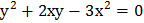# If the pairs of linesandhave exactly one line in common, then the joint equation of the other two lines is given by a)b)c)d)## Question ID - 52928 :- If the pairs of linesandhave exactly one line in common, then the joint equation of the other two lines is given by a)b)c)d)3537

(b)

Letbe a line common to the given pairs of lines. ThenandandorBut for, the two pairs have both the lines common. Soand the slopeof the line common to both the pairs is 1. Now,andso the equation of the required lines isNext Question :
 If two vertices of a triangle areand, orthocentre lies at the origin and centroid on the line, then the third vertex lies at a) (7, 4) b) (8, 14) c) (12, 21) d) None of these